Electrolysis is defined as a process of decomposition of electrolytes in aqueous or molten state by passing electric current through it.

The process of electrolysis is carried out in electrolytic cell. Electrolytic cell consists of two electrodes and an electrolyte. When electricity is passed through aqueous solution of electrolyte, the cations of the electrolyte are reduced at cathode and anions are oxidized at anode.

Let us take an example of a simple electrolytic cell consisting of two copper strips dipped in a copper sulphate solution. When electricity is passed through the solution, Cu^2+ ions discharge at cathode and copper metal is deposited.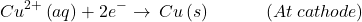At the anode, Copper is converted into Copper ions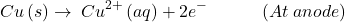Calculation of the charge(or electricity) consumed in the electrolytic cell:

The charge passed through the electrolytic cell is equal to the product of current and time.The amount of charge required for the oxidation or reduction depends on the stoichiometry of the electrolyte. For example in the above reaction,Here two moles of electrons is required for the reduction of copper ions.

We know charge on 1 electron=So, charge on 1 mol of electron=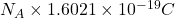==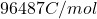This quantity of electricity is called Faraday (). So, for the deposition of Copper, two mol of electrons or 2F charge is required. By knowing the amount of electricity or charge passed, we can calculate the mass of the atom deposited.

Let us understand the concept of charge in electrolysis more clearly while solving a numerical problem through this video.

This video covers the solution of: Lead oxide(IV) is reduced to the cathode of lead ion(II) electrochemical cell with a current of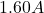for the duration of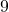hours and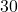minutes. Calculate the mass of lead(IV) oxide that has been consumed.